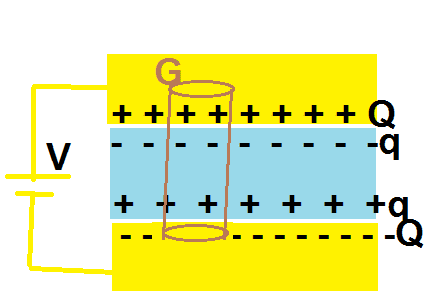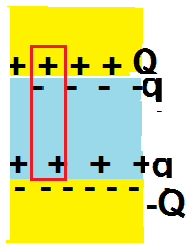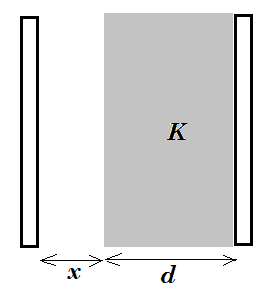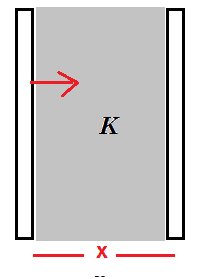# Force between the plates of a capacitor with dielectric slab inserted

Pushoam
Homework Statement:
The electric slab is inserted between the plates of an isolated capacitor. The force between the plates will
a) increase b) decrease
c) remain unchanged d)become zero.
Relevant Equations:
$$\vec E = \frac {\vec E_0} K$$
The the electric field inside decreases due to the presence of a dielectric by a factor of dielectric constant K. Hence the force between the plates will decrease.

Is this right?

Homework Helper
Gold Member
The field inside the dielectric, ##\frac {E_0}{K}##, is not the field that determines the force on a plate. It might help to draw a picture showing the distribution of free charge on the plates and the induced charge on the dielectric. The net force acting on the free charge of one plate is due to the charge on the other plate and the induced charge on the dielectric.

•Pushoam
PushoamTaking the cylindrical surface as Gaussian surface and applying Gauss's law, electric field at each plate is unaffected by the presence of dielectric. Hence, the force between the plates remain unchanged.

It also illustrates that the field due to dielectric outside the dielectric is 0 here.

•TSny
Homework Helper
Gold Member
View attachment 321943
Taking the cylindrical surface as Gaussian surface and applying Gauss's law, electric field at each plate is unaffected by the presence of dielectric. Hence, the force between the plates remain unchanged.

It also illustrates that the field due to dielectric outside the dielectric is 0 here.
Looks good.

Pushoam
Looks good.
Thank you.

Homework Helper
Gold Member
View attachment 321943
Taking the cylindrical surface as Gaussian surface and applying Gauss's law, electric field at each plate is unaffected by the presence of dielectric. Hence, the force between the plates remain unchanged.

It also illustrates that the field due to dielectric outside the dielectric is 0 here.
The Gaussian surface that you have chosen encloses zero net charge and the normal component of the electric field is zero on its faces. So Gauss's law says 0 = 0 which is always true and does not help. The field at each plate is the same as the field at a point inside the dielectric. Therefore, if you want to make a Gauss's law argument, one of the faces must be inside the dielectric and parallel to the plates, but then you will have to figure out the enclosed charge.

My personal opinion is that Gauss's law is not a good way to approach this problem. The clean way to do this is by using an energy argument. Note that the force between plates can be found from ##F=-\dfrac{\partial U}{\partial x}## where ##U## is the potential energy stored in the capacitor and ##x## is the separation between plates. Calculate the force with and without the dielectric and compare. Also note that the disembodied hand that inserts the dielectric (presumably at constant speed) does external work on the dielectric + charged capacitor system therefore the potential energy and hence the force cannot stay the same.

•nasu and Pushoam
Homework Helper
Gold Member
The Gaussian surface that you have chosen encloses zero net charge and the normal component of the electric field is zero on its faces.
I was thinking that the Gaussian surface does not enclose the negative free charge on the bottom plate. Maybe @Pushoam can clarify.

Last edited:
Homework Helper
Gold Member
I was thinking that the Gaussian surface does not include the negative free charge on the bottom plate. Maybe @Pushoam can clarify.
I went by the drawing that shows the bottom Gaussian surface in the yellow region of the conductor. As long as that is the case, the field at the surface is zero. If the bottom Gaussian surface at the interface between conductor and dielectric, the electric field is discontinuous right at the surface.

I don't think that it is possible to have a Gaussian surface bottom face inside the conductor and at the same time exclude the negative free charge which is right at the interface between the conductor and the dielectric. If the bottom Gaussian surface is right at the interface, is the free charge enclosed or not enclosed? Is the electric field, which is discontinuous right there, zero or non-zero?

Homework Helper
The capacitor is connected to a battery. You don't need Gauss' law to show that the field inside the capacitor stays the same. Is is just ##\Delta V= E d## and neither voltage nor distance change when you introduce the dielectric. But the density of the free charges on the plates increases when you introduce the dielectric so the force betwen the plates should increase. The same can de shown by by taking two capacitors with same geometry and same potential difference, one with dielectric and one without, and calculate the force by the energy method sugegsted by Kuruman, for each case.

The situation regarding the field is different if the capacior is not connected while the dielectric is introduced. The electric potential decreases and so does the field between the plates.

•Pushoam
Homework Helper
Gold Member
The capacitor is connected to a battery.
The problem statement says that the capacitor is isolated.

•Pushoam
Homework Helper
Gold Member
I went by the drawing that shows the bottom Gaussian surface in the yellow region of the conductor. As long as that is the case, the field at the surface is zero. If the bottom Gaussian surface at the interface between conductor and dielectric, the electric field is discontinuous right at the surface

I don't think that it is possible to have a Gaussian surface bottom face inside the conductor and at the same time exclude the negative free charge which is right at the interface between the conductor and the dielectric. If the bottom Gaussian surface is right at the interface, is the free charge enclosed or not enclosed? Is the electric field, which is discontinuous right there, zero or non-zero?
I think it doesn't really change anything if you imagine a very thin layer of vacuum separating the dielectric from the bottom plate in the figure of post #3. The bottom surface of the Gaussian surface is in this vacuum layer, not in the conducting material of the plate.

Using the energy approach, if you pull one plate away from the dielectric by a distance ##x##, the increase in potential energy is equal to the electric field energy created in the vacuum space of thickness ##x## between the dielectric and the plate. The field in this space is the same as the field that would exist between the plates without the dielectric. So, the increase in potential energy is independent of the presence of the dielectric.

•Pushoam
Homework Helper
Gold Member
I think it doesn't really change anything if you imagine a very thin layer of vacuum separating the dielectric from the bottom plate in the figure of post #3. The bottom surface of the Gaussian surface is in this vacuum layer, not in the conducting material of the plate.
Perhaps you might consider sending me your solution via PM and I will send you mine. Who knows? Maybe we will find ourselves in agreement.

Homework Helper
Gold Member
Perhaps you might consider sending me your solution via PM and I will send you mine. Who knows? Maybe we will find ourselves in agreement.
OK. Sounds good.

Homework Helper
The problem statement says that the capacitor is isolated.
The diagram with the Gaussian shows a battery connected. But you are right, the statement says it is isolated.

•Pushoam and TSny
Homework Helper
Gold Member
The diagram with the Gaussian shows a battery connected.
I didn’t notice the battery in the diagram. It shouldn’t be there. Thanks.

•Pushoam
Pushoam
I was thinking that the Gaussian surface does not enclose the negative free charge on the bottom plate. Maybe @Pushoam can clarify.

Yes, this shows that electirc field on the bottom plate depends on the charge of the top plate only.

Pushoam
I went by the drawing that shows the bottom Gaussian surface in the yellow region of the conductor. As long as that is the case, the field at the surface is zero. If the bottom Gaussian surface at the interface between conductor and dielectric, the electric field is discontinuous right at the surface.

I don't think that it is possible to have a Gaussian surface bottom face inside the conductor and at the same time exclude the negative free charge which is right at the interface between the conductor and the dielectric. If the bottom Gaussian surface is right at the interface, is the free charge enclosed or not enclosed? Is the electric field, which is discontinuous right there, zero or non-zero?
The free charge at the bottom plate is not included and the field there is non-zero. That's why there is force on the bottom plate due to top plate.

Homework Helper
Gold Member
The free charge at the bottom plate is not included and the field there is non-zero. That's why there is force on the bottom plate due to top plate.
I am not sure that I can visualize this. Can you post a fresh picture? Please draw the Gaussian cylinder as a rectangle and we will understand what sides are the flat surfaces. Remember that the bound charges are right up against the free charges at the interface between conductor and dielectric. Thanks.

Last edited:
Pushoam
I am not sure that I can visualize this. Can you post a fresh picture? Please the Gaussian cylinder as a rectangle and we will understand what sides are the flat surfaces. Remember that the bound charges are right up against the free charges at the interface between conductor and dielectric. Thanks.
I have to calculate force on the bottom plate. So, I need to calculate electric field acting on the facing surface charge of the bottom plate. So, I took my bottom Gaussian surface near the bottom surface charge but not including it (as if I include the bottom charge, then the total enclosed charge becomes 0 and it doesn't serve the purpose.).

Homework Helper
Gold Member
I have to calculate force on the bottom plate. So, I need to calculate electric field acting on the facing surface charge of the bottom plate. So, I took my bottom Gaussian surface near the bottom surface charge but not including it (as if I include the bottom charge, then the total enclosed charge becomes 0 and it doesn't serve the purpose.).
Like I said, I cannot picture this from a word description.. Please post a picture of the entire Gaussian surface that you have chosen.

Homework Helper
If the capacitor is disconnected from the battery, the (free) charge on the plates stays the same and so does the free charge density. The field of the free charges does not change. And the field outside the polarized slab of dielectric is zero so it has no effect on the plates.
When they say the force "between the plates" I suppose that they mean the force due to the mutual attraction between the plates. This will not change as both the charge on the plates and the distance stays the same.
If instead it means the force on one of the plates due to interactions with all the rest, this ideally stays the same too because the field of the dielectric outside its surface is zero. Of course, this is so for infinite slab. But all the capacitor problems work in the approximation of neglecting edge effects.

Homework Helper
I have to calculate force on the bottom plate.
The question asks for the force between the plates. This is not the same as the total force acting on one plate. The force between me and the Earth is not the same as the force acting on me at this time. Or any other time when I am not in free fall in a vacuum.
It may not make a difference for this problem but in general the two things are different.

Pushoam
Considering the isolated capacitor,
Case 1: When there is no dielectric,$$U = \frac {Q^2} {2C} = \frac {Q^2 x} {2A \epsilon_0}$$$$F = - \frac {d\frac {Q^2 x} {2A \epsilon_0}} {dx} = \frac {-Q^2} {2A \epsilon_0},$$ where "-" sign shows that the conservative force between the plates is attractive i.e. each plate is attracted to constitute the system by a force of magnitude |F| .

Case2: When there is dielectric inserted between the plates,
Due to conservation of energy, the charge distribution takes place in such a way that the U(x) remains same although C changes (as the system is isolated). Hence, $$U(x) = Mx, M = \frac {Q^2 } {2A \epsilon_0}, \Rightarrow F =\frac {-Q^2} {2A \epsilon_0}$$
Now, as the potential energy belongs to the system consisting of capacitor with inserted dielectric, the plates as well as the dielectric is attracted by a conservative force of magnitude |F|.
Hence, the force is unchanged.

Homework Helper
Gold Member
This will not change as both the charge on the plates and the distance stays the same.
My argument is that the bound charges at one conductor-dielectric interface have opposite sign to the free charges effectively partially reducing the surface charge density that the charges at the opposing interface "see". This reduces the attraction between plates.

The energy argument illustrates this better. If a dielectric is inserted at constant charge, the energy stored in the capacitor is reduced by a factor of ##\kappa.## Reduced stored potential energy means reduced force that one plate exerts on the other. This can be verified by a simple thought experiment. Release the plates and let them collide. The plates in the configuration of higher potential energy will have higher kinetic energy before they collide which means higher acceleration and hence larger force.

The question asks for the force between the plates.
I find such questions ambiguous. Forces are exerted by entity A on entity B. This format clearly identifies the system and the external force that acts on it.

Homework Helper
Gold Member
Considering the isolated capacitor,
Case 1: When there is no dielectric,$$U = \frac {Q^2} {2C} = \frac {Q^2 x} {2A \epsilon_0}$$$$F = - \frac {d\frac {Q^2 x} {2A \epsilon_0}} {dx} = \frac {-Q^2} {2A \epsilon_0},$$ where "-" sign shows that the conservative force between the plates is attractive i.e. each plate is attracted to constitute the system by a force of magnitude |F| .

Case2: When there is dielectric inserted between the plates,
Due to conservation of energy, the charge distribution takes place in such a way that the U(x) remains same although C changes (as the system is isolated). Hence, $$U(x) = Mx, M = \frac {Q^2 } {2A \epsilon_0}, \Rightarrow F =\frac {-Q^2} {2A \epsilon_0}$$
Now, as the potential energy belongs to the system consisting of capacitor with inserted dielectric, the plates as well as the dielectric is attracted by a conservative force of magnitude |F|.
Hence, the force is unchanged.
Case 1 is fine. That's what I got.
Case 2 is a Procrustean attempt to fit your derivation to what you are convinced is the case. Just put in the changed capacitance in the expression for the potential energy, follow the same steps as in Case 1 and see what you get. If you don't like the result, check you calculations for mistakes. If you find no mistakes, you must accept the result. Then try to find reasons why this unanticipated result makes sense while your preconceptions did not.

Pushoam
The question asks for the force between the plates.
Potential energy is defined of a system. So, when we differentiate it w.r.t. -x, we get the force F required to constitute the system. That means a force of magnitude |F| acts on each constituting part in a direction such that the parts are attracted to constitute the system.
For example, here, each plate attracts the other with a force of magnitude |F|.

Staff Emeritus
Homework Helper
My argument is that the bound charges at one conductor-dielectric interface have opposite sign to the free charges effectively partially reducing the surface charge density that the charges at the opposing interface "see". This reduces the attraction between plates.
The charges on the top plate are partially shielded by the surface charge at the top of the dielectric, but the bottom plate also sees the surface charges on the bottom of the dielectric. Consequently, the force would remain the same with or without the dielectric present.

Pushoam
Case 2 is a Procrustean attempt to fit your derivation to what you are convinced is the case. Just put in the changed capacitance in the expression for the potential energy, follow the same steps as in Case 1 and see what you get. If you don't like the result, check you calculations for mistakes. If you find no mistakes, you must accept the result. Then try to find reasons why this unanticipated result makes sense while your preconceptions did not.
I am not trying to fit the answer.
Case 2:
$$U = \frac {Q^2} {2KC} = \frac {Q^2 x} {2KA \epsilon_0}$$ where K is the dielectric constant.
This gives ## F = \frac {-Q^2} {2KA \epsilon_0}##.
But, the system is isolated and the potential energy is defined of a system (which here contains dielectric + plates). And conservation of energy applies that potential energy does not change. Hence, I concluded that as the capacitance gets multiplied by K, so the charge on the capacitor, too, changes such that potential energy remains constant. That's why in post # 23, I put U as Mx and then wrote that M is equal to ## \frac{Q^2}{2A\epsilon_0}##. It's like,
$$\text{U in case 1 = U in case 2 }$$$$\frac {Q^2x} {2A\epsilon_0} = Mx$$ $$\frac{Q^2}{2A\epsilon_0} =M$$

Pushoam
The energy argument illustrates this better. If a dielectric is inserted at constant charge, the energy stored in the capacitor is reduced by a factor of ##\kappa.## Reduced stored potential energy means reduced force that one plate exerts on the other.
I got that the charge on the plate can't be changed as the capacitor is isolated and charge is conserved. Hence, in case 2: ##F = \frac {-Q^2} {2KA \epsilon_0}##.
But, then what happens to the change in potential energy?

Homework Helper
Gold Member
I got that the charge on the plate can't be changed as the capacitor is isolated and charge is conserved. Hence, in case 2: ##F = \frac {-Q^2} {2KA \epsilon_0}##.
But, then what happens to the change in potential energy?
The dielectric + capacitor system is not isolated. The disembodied hand that inserts the dielectric, presumably at constant speed, has to hold it back from being sucked in and thus does negative work on the system.

•Pushoam
Pushoam
Like I said, I cannot picture this from a word description.. Please post a picture of the entire Gaussian surface that you have chosen.Here is the Gaussian surface I was talking of. It includes charge of upper plate and upper part and lower part of dielectric. Electric field due to the dielectric outside the dielectric is 0. Hence, the electric field at bottom plate (which is outside the dielectric) is only due to upper plate, not the dielectric.

The bound charges and the free charges are not mixed with each other. These are divided by a surface (which is invisible here as I cannot draw it here). The bottom part of my Gaussian surface is here.
Now, as this surface is close to the facing surface of bottom plate, the electric field on this surface is close to the electric field acting on the charges of bottom plate.

Just above the bottom part of Gaussian surface, ## \vec E = \frac{\vec E_0}K## and just below the bottom part of Gaussian surface, ## \vec E = \vec E_0 ##. The bottom plate is just below, hence here, ## \vec E = \vec E_0 ##. The bottom part of Gaussian surface is at the point of discontinuity.

This approach shows that the force remains same in both cases (1) and (2). Which mistake am I making in this approach?

Homework Helper
Gold Member
Here's another way to argue that the force is the same with or without the dielectric. A standard problem in the textbooks is to work out an expression for the capacitance ##C## of a parallel plate capacitor with a dielectric slab that doesn't fill the space between the plates.I believe the answer is $$\frac 1 C = \frac{1}{\varepsilon_0 A}\left( x + \frac{d}{K}\right)$$ where ##A## is the plate area.

So, when the capacitor is charged with ##\pm Q## on the plates, the energy stored is $$U = \frac{Q^2}{2C} = \frac{Q^2}{2\varepsilon_0A}\left(x + \frac d K \right)$$

Moving the left plate to vary ##x##, ##U## will change. The magnitude of the force on the left plate is then $$|F| = \frac {dU}{dx} = \frac{Q^2}{2\varepsilon_0 A}$$ which is independent of ##x, d, ## and ##K##. So this will be the force on the left plate in the limit of ##x \rightarrow 0## and the force will be independent of ##K##.

•nasu and Pushoam
Pushoam
I think there is a thin layer of vacuum between the dielectric and the plate. This thin layer doesn't matter while calculating potential energy of the system (capacitor + dielectric) as integral of the force over this small distance (leading to thinness of the layer) is negligible. But, it does matter while calculating force as the electric field in this layer is unaffected by the presence of dielectric.

The expression for force which @TSny has got is calculated in the region without dielectric (here ## x\rightarrow 0## means that the plate goes close to the dielectric, yet it is slightly out of the dielectric, not touching it. So, here d acts as a constant and gets out of differentiation.Instead of putting the plate at a distance x from dielectric in vacuum and then differentiating its potential energy w.r.t. x, let's try to move the plate inside the dielectric (which is possible when the dielectric is fluid).
Here, ## U = \frac{Q^2}{2A\epsilon_0}\frac{x}{K}, F = {-\frac{Q^2}{2KA\epsilon_0}}##

But, according to the question, the dielectric is a slab and hence, the plate cannot move inside the dielectric causing "d" to act as a constant and there is a thin layer of vacuum between the slab and the dielectric, which corresponds to ## F = -\frac{Q^2}{2A\epsilon_0}## satisfied by both approaches (potential energy and Gauss's law).

Last edited: